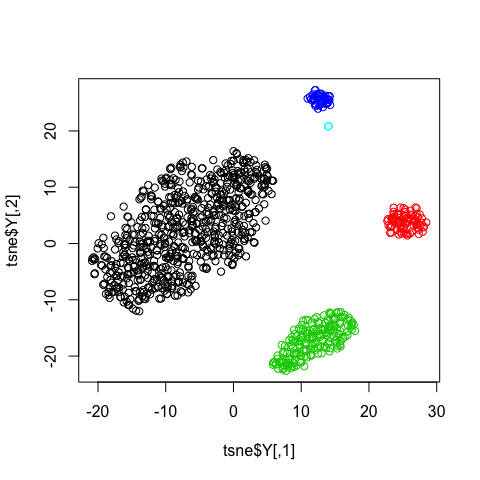# A quick tour of HDclust

## Introduction

HDclust is a contributed R package for clustering high dimensional data using Hidden Markov model on Variable Blocks (HMM-VB). Briefly, the model consists of sequentially dependent groups of variables , i.e., the variable blocks. Each variable block follows a Gaussian mixture distribution. Statistical dependence among the blocks is modelled by the Markov process of the underlying states (i.e. the mixture component identities). The Baum-Welch (BW) estimation algorithm is used to fit the model to data. The clustering is performed by finding the density modes with Modal Baum-Welch algorithm (MBW). In the case of a single variable block the model reduces to a regular Gaussian Mixture Model (GMM). The package provides functions to train HMM-VB model with known or unknown variable block structure; search for the optimal number of states of HMM-VB model using BIC; cluster data with trained HMM-VB model; and align clustering results for different data sets using the same HMM-VB model.

To illustrate HDclust functionality we will use a standard R data set “faithful” from package ‘datasets’, and two synthetic data sets sim2 and sim3. sim2 is a 5-dimensional data set with 5000 data points. Samples were drawn from a 10-component Gaussian Mixture Model (GMM). By specific choice of the means, the data contains 10 distinct clusters. sim3 is a 40-dimensional data set. The first 10 dimensions were generated from a 3-component Gaussian Mixture Model (GMM). The remaining 30 dimensions were generated from a 5-component GMM. By specific design of the means, covariance matrices and transition probabilities, the data contain 5 distinct clusters. For more details on sim2 and sim3 see the reference.

library(HDclust)

## Define a variable block structure

Dependence among the groups of variables is defined in a variable block structure. When there is only a single variable block in the structure, the model reduces to a regular GMM. Note, however, that clustering is performed by finding density modes, so results will be different from traditional GMM clustering.

# variable structure with one variable block of 3 variables and two mixture components, which corresponds to GMM model
Vb<- vb(1, dim=3, numst=2)
show(Vb)
#> ------------------------
#> Variable block structure
#> ------------------------
#>
#> Data dimensionality = 3
#>
#> Number of variable blocks = 1
#>
#> Dimensionality of variable blocks: 3
#>
#> Number of states in variable blocks: 2
#>
#> Variable order in variable blocks:
#> Block 1 : 1 2 3
#> ------------------------

In case of the variable block structure with more than one block, definition becomes slightly more complicated. A user has to provide dimensionality, number of mixture components and variable order for each variable block:

# variable structure with two blocks. Dimensionality of data is 40. First block contains 10 variable with 3 mixture components. Second block has 30 variables with 5 mixture components. Variable order is natural.
Vb <- vb(2, dim=40, bdim=c(10,30), numst=c(3,5), varorder=list(c(1:10),c(11:40)))
show(Vb)
#> ------------------------
#> Variable block structure
#> ------------------------
#>
#> Data dimensionality = 40
#>
#> Number of variable blocks = 2
#>
#> Dimensionality of variable blocks: 10 30
#>
#> Number of states in variable blocks: 3 5
#>
#> Variable order in variable blocks:
#> Block 1 : 1 2 3 4 5 6 7 8 9 10
#> Block 2 : 11 12 13 14 15 16 17 18 19 20 21 22 23 24 25 26 27 28 29 30 31 32 33 34 35 36 37 38 39 40
#> ------------------------

## Model selection

While dependence among the groups of variables can be inferred using the domain knowledge, it is hard to predict the number of mixture states in each variable block. hmmvbBIC() can search for an optimal number of states in the variable block structure. A user needs to provide all parameters of the variable block structure for which the search will be performed. The argument numst in variable block structure provided to hmmvbBIC() will be ignored during the search. The search minimizes Bayesian information criterion (BIC) and provides two options for the user.

By default the search changes the number of states in the variable blocks and estimates BIC for each model. In this case, number of states is kept the same for all variable blocks.

# by default number of states in each block is varied from 1 to 9
data("faithful")
Vb <- vb(1, dim=2, numst=1)
set.seed(12345)
modelBIC <- hmmvbBIC(faithful, VbStructure=Vb)
show(modelBIC)
#> ------------------------------------------------------
#> Optimal number of states: 2 with BIC: 2322.201
#> ------------------------------------------------------

Model selection results can be visualized in the following way:

plot(modelBIC, xlab='# mixture components per block', ylab='BIC')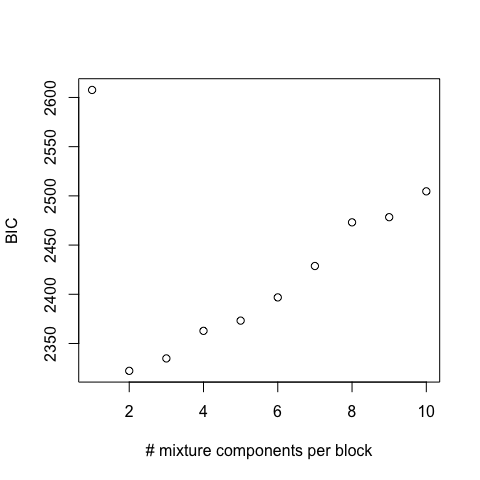Second option is to provide a list with configurations for the number of states in variable blocks. In this case, the algorithm computes BIC for each configuration and estimates the optimal configuration as the one with the smallest BIC value.

# user-provided configurations for the number of states in each block
data("sim3")
Vb <- vb(2, dim=40, bdim=c(10,30), numst=c(1,1), varorder=list(c(1:10),c(11:40)))
set.seed(12345)
configs = list(c(1,2), c(3,5))
modelBIC <- hmmvbBIC(sim3[,1:40], VbStructure=Vb, configList=configs)
show(modelBIC)
#> ------------------------------------------------------
#> Optimal configuration: 3 5 with BIC: -2976.672
#> ------------------------------------------------------

In both cases, the output is an object of class HMMVBBIC that contains HMM-VB parameters for the optimal configuration. The optimal HMM-VB can be accessed with getOptHMMVB method.

If you want to see more information like loglikelihood for each data point, you can use a get function like getLoglikehd().

# we just illustrate the loglikelihood of the first 10 data points beacause the data set is huge
getLoglikehd(modelBIC)[1:10]
#>    18.0329772   9.8358373  13.6714102  14.9280804   0.4970656
#>    15.9723222 -10.3004831  13.7017806  15.5448324   7.4448152

## Train an HMM-VB model

There are two ways to train an HMM-VB model. One way is to use a variable block structure motivated by domain knowledge.

data("sim3")
set.seed(12345)
Vb <- vb(2, dim=40, bdim=c(10,30), numst=c(3,5), varorder=list(c(1:10),c(11:40)))
hmmvb <- hmmvbTrain(sim3[1:40], VbStructure=Vb)
show(hmmvb)
#> --------------------------------------
#> Hidden Markov Model on Variable Blocks
#> --------------------------------------
#>
#> ------------------------
#> Variable block structure
#> ------------------------
#>
#> Data dimensionality = 40
#>
#> Number of variable blocks = 2
#>
#> Dimensionality of variable blocks: 10 30
#>
#> Number of states in variable blocks: 3 5
#>
#> Variable order in variable blocks:
#> Block 1 : 1 2 3 4 5 6 7 8 9 10
#> Block 2 : 11 12 13 14 15 16 17 18 19 20 21 22 23 24 25 26 27 28 29 30 31 32 33 34 35 36 37 38 39 40
#> ------------------------
#>
#> BIC = -8939.257
#>
#> Covariance matrices are diagonal = FALSE
#>
#> To show parameters of HMMs, access elements in HmmChain list.

Parameters of trained HMM-VB can be accessed by get functions:

Vb <- getVb(hmmvb) # variable block structure
hmmChain <- getHmmChain(hmmvb) # list with HMM models
diagCov <- getDiagCov(hmmvb) # indicator whether covariance matrices are diagonal
bic <- getBIC(hmmvb) # BIC value

# below we show HMM-VB parameters for the first variable block
numst <- getNumst(hmmChain[]) # number of mixture components in variable block
prenumst <- getPrenumst(hmmChain[]) # number of mixture components in the previous variable block
hmmParam <- getHmmParam(hmmChain[]) # list with priors, transition probabilities, means, covariance matrices and other parameters of all states of HMM

## Train an HMM-VB model with unknown variable block structure

If a variable block structure is unknown, one can search for it with hmmvbTrain() function. The search is performed by a greedy algorithm. Thus multiple permutations of variables are needed for the best estimation of the variable block structure. With many permutations, the search becomes a time-consuming process, and to accelerate it, we advice to use as many cores as your machine has. This is done with parameter nthread, which we set to 4 in the example below, since our machine has 4 cores.

data("sim2")
set.seed(12345)
hmmvb <- hmmvbTrain(sim2[1:5], searchControl=vbSearchControl(nperm=5), nthread=4)
#>
#> More than one thread is used with OpenMP not configured. Make sure your compiler supports OpenMP and reinstall the package
show(hmmvb)
#> --------------------------------------
#> Hidden Markov Model on Variable Blocks
#> --------------------------------------
#>
#> ------------------------
#> Variable block structure
#> ------------------------
#>
#> Data dimensionality = 5
#>
#> Number of variable blocks = 1
#>
#> Dimensionality of variable blocks: 5
#>
#> Number of states in variable blocks: 12
#>
#> Variable order in variable blocks:
#> Block 1 : 1 2 3 4 5
#> ------------------------
#>
#> BIC = 25014.09
#>
#> Covariance matrices are diagonal = FALSE
#>
#> To show parameters of HMMs, access elements in HmmChain list.

Suggested variable block structure has a single variable block. That is in hand with the data, which was generated from a mixture of 10 Gaussian components. After finding the variable block structure, we recommend to run model selection to refine the number of mixture components:

modelBIC <- hmmvbBIC(sim2[1:5], VbStructure=getVb(hmmvb), numst=1:15, nthread=4)
show(modelBIC)
#> ------------------------------------------------------
#> Optimal number of states: 11 with BIC: 24849.79
#> ------------------------------------------------------

## Clustering with HMM-VB

After training the HMM-VB model we can perform data clustering. If variable block structure is known, we can start with model selection to refine number of mixture components in variable blocks. The output object of type HMMVBBIC is then used in function hmmvbClust() with argument bicObj to perform clustering.

data("faithful")
VbStructure <- vb(nb=1, dim=2, numst=1)
set.seed(12345)
modelBIC <- hmmvbBIC(faithful, VbStructure)
clust <- hmmvbClust(faithful, bicObj=modelBIC)
show(clust)
#> ------------------------------------------------------
#> Clustering with Hidden Markov Model on Variable Blocks
#> ------------------------------------------------------
#>
#> Number of clusters = 2
#>
#> Cluster sizes: 97 175
plot(faithful[,1], faithful[,2], xlab='eruptions', ylab='waiting', col=getClsid(clust))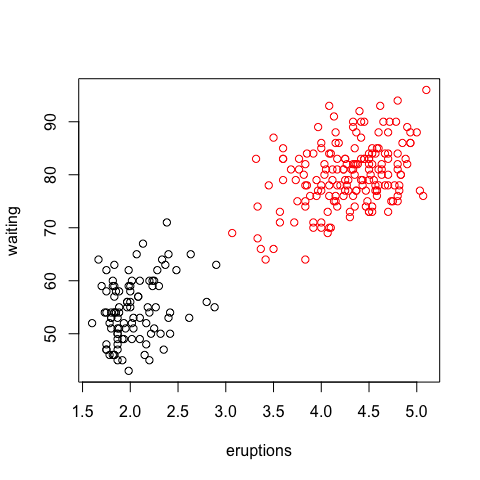hmmvbClust() returns an object of type HMMVBclust that contains the clustering results, including Viterbi sequences and cluster modes.

clustParam <- getClustParam(clust)
mode <- clustParam$mode # a matrix with cluster modes vseq <- clustParam$vseq # A list with integer vectors representing distinct Viterbi sequences for the dataset

If variable block structure is unknown, we first search for it, then refine number of mixture components with model selection, and perform the clustering.

# If variable block structure is unknown
data("sim2")
set.seed(12345)

# find variable block structure
hmmvb <- hmmvbTrain(sim2[,1:5], searchControl=vbSearchControl(nperm=5), nthread=4)

# refine number of states in variable block structure by model selection
modelBIC <- hmmvbBIC(sim2[,1:5], VbStructure=getVb(hmmvb), numst=1:15, nthread=4)
clust <- hmmvbClust(data=sim2[,1:5], bicObj=modelBIC)
show(clust)
#> ------------------------------------------------------
#> Clustering with Hidden Markov Model on Variable Blocks
#> ------------------------------------------------------
#>
#> Number of clusters = 10
#>
#> Cluster sizes: 109 500 508 1136 504 753 502 485 49 454

Clustering results can also be plotted using two visualization algorithms.

By default results will be plotted using t-SNE algorithm from Rtsne package.

palette(c(palette(), "purple", "brown")) # extend palette to show all 10 clusters
plot(clust)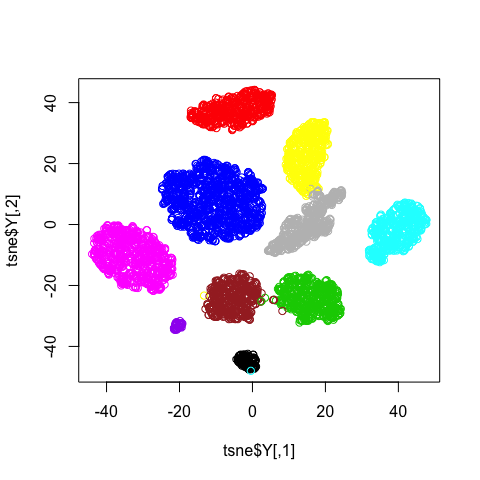Results can also be shown in 2 dimensional space reduced by PCA:

plot(clust, method='PCA')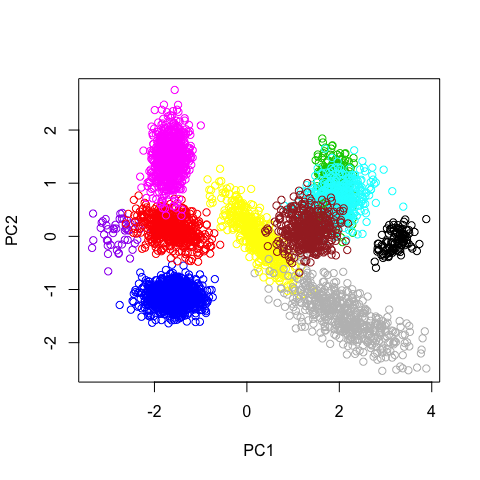## Cluster alignment

Imagine that you have already performed clustering with HMM-VB for one data set and now another similar data set is available. One can use existing HMM-VB model and align cluster labels of the second data set with the clusters obtained for the first data set:

data("sim3")

# split data set in two halves
X1 = sim3[1:500,]
X2 = sim3[501:1000,]

set.seed(12345)
Vb <- vb(2, 40, c(10,30), c(3,5), list(c(1:10),c(11:40)))

# train HMM-VB on dataset X1 and cluster data
hmmvb <- hmmvbTrain(X1[1:40], VbStructure=Vb)
clust1 <- hmmvbClust(X1[1:40], model=hmmvb)
show(clust1)
#> ------------------------------------------------------
#> Clustering with Hidden Markov Model on Variable Blocks
#> ------------------------------------------------------
#>
#> Number of clusters = 5
#>
#> Cluster sizes: 341 27 80 26 26

# cluster data set X2 using HMM-VB and cluster parameters for dataset X1
clust2 <- hmmvbClust(X2[1:40], model=hmmvb, rfsClust=getClustParam(clust1))
#> All found viterbi sequences match with sequences in reference clusters

show(clust2)
#> ------------------------------------------------------
#> Clustering with Hidden Markov Model on Variable Blocks
#> ------------------------------------------------------
#>
#> Number of clusters = 5
#>
#> Cluster sizes: 346 17 103 13 21

If you also want to compute the loglikelihood for the new data set, it can be realized by setting the parameter getlikelh to be TRUE in clustControl():

clust2 <- hmmvbClust(X2[1:40], model=hmmvb, rfsClust=getClustParam(clust1), control=clustControl(getlikelh = TRUE))
#> All found viterbi sequences match with sequences in reference clusters
# we just illustrate the loglikelihood of the first 10 data points beacause the data set is huge
getLoglikehd(clust2)[1:10]
#>     17.653071    9.835956   16.587321 -476.377336   22.434999
#>     20.201192   19.094334   13.725819    7.412597    4.031532

## Solution for slow data clustering with many changes in mode merging parameters

If data clustering takes a significant amount of time and many changes in mode merging parameters are required to achieve the desired clustering result, we recommend to use hmmvbFindModes() and clustModes(). Function hmmvbFindModes() finds all density modes in the data, and clustModes() clusters the modes using hierarchical clustering. Under the hood clustModes() uses dist(), hclust() and cutree() calls from the package stats, and a user can specify parameters for all of them with dist.args, hclust.args and cutree.args arguments.

data("sim3")

set.seed(12345)
Vb <- vb(2, 40, c(10,30), c(3,5), list(c(1:10),c(11:40)))

# train HMM-VB
hmmvb <- hmmvbTrain(sim3[1:40], VbStructure=Vb)

# find all density modes
modes <- hmmvbFindModes(sim3[1:40], model=hmmvb)
show(modes)
#> ------------------------------------------------------
#> Clustering with Hidden Markov Model on Variable Blocks
#> ------------------------------------------------------
#>
#> Number of clusters = 5
#>
#> Cluster sizes: 682 83 183 46 6

# cluster density modes
mergedModes <- clustModes(modes, cutree.args = list(h=1.0))

show(mergedModes)
#> ------------------------------------------------------
#> Clustering with Hidden Markov Model on Variable Blocks
#> ------------------------------------------------------
#>
#> Number of clusters = 5
#>
#> Cluster sizes: 682 83 183 46 6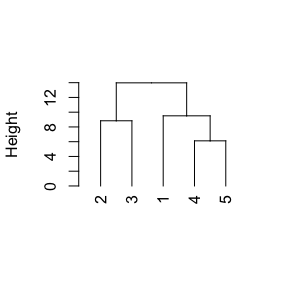plot(mergedModes)